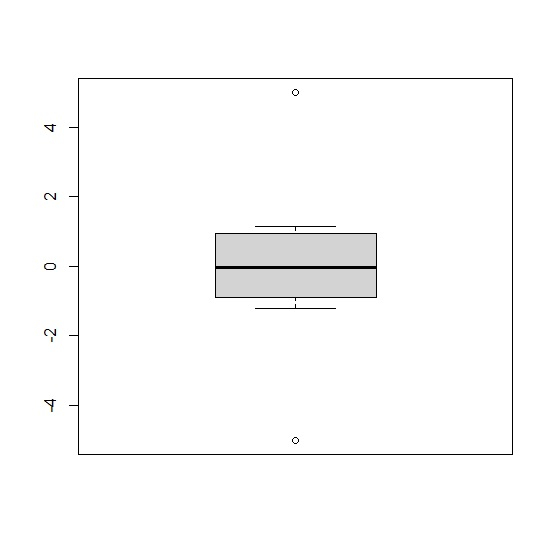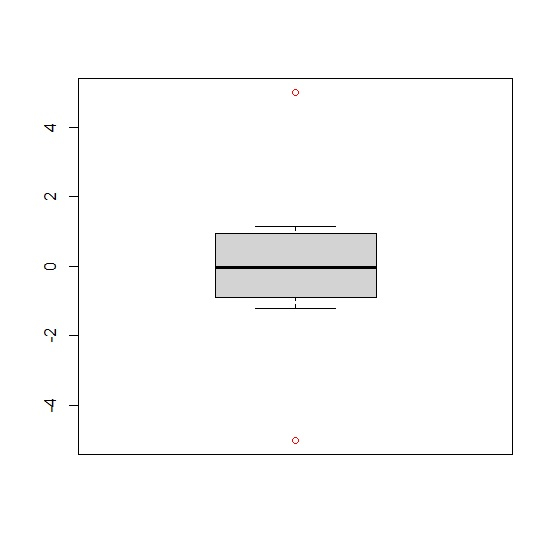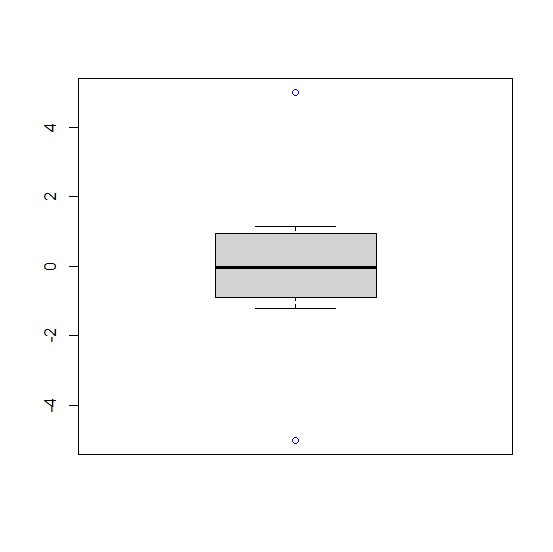# How to change the color of outliers in base R boxplot?

To change the color of outliers in base R boxplot, we can use outcol argument in boxplot function.

For example, if we have a vector called X that contains some outliers then we can create a boxplot of X with blue color outliers by using the below given command −

boxplot(X,outcol="red")

## Example

To change the color of outliers in base R boxplot, use the code given below: −

x<-c(-5,rnorm(5),5)
boxplot(x)

## Output

If you execute the above given code, it generates the following output −To change the color of outliers in base R boxplot, use the following code −

x<-c(-5,rnorm(5),5)
boxplot(x,outcol="red")

## Output

If you execute the above given code, it generates the following output −To change the color of outliers in base R boxplot, use the following code −

x<-c(-5,rnorm(5),5)
boxplot(x,outcol="blue")

## Output

If you execute the above given code, it generates the following output −